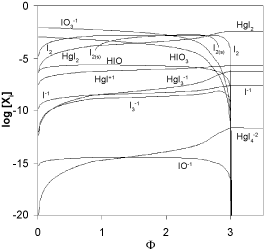Figure 3: The plots for different iodine species in the system KIO3 (C0=0.01 mol/L) + HCl (Ca=0.02 mol/L) + H2SeO3 (CSe=0.02 mol/L) + HgCl2 (CHg=0.07 mol/L) titrated with V mL of C6H8O6 (C=0.1 mol/L) .
Goto home»